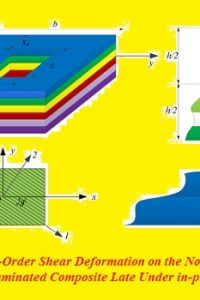اسم المؤلف
Dr. Haider K. Ammash
التاريخ
5 مارس 2021
المشاهدات
126
التقييم(لا توجد تقييمات)Loading...

بحث بعنوان
Effect of Higher-Order Shear Deformation on the Nonlinear Dynamic Analysis of Laminated Composite Late Under in-plane Loads
Dr. Haider K. Ammash
Iraq , Al-Qadissiya University , Engineering College ,Civil
Engineering
Keywords: Dynamic analysis, Nonlinear analysis, Composite plate, Finite element method
Abstract: A nonlinear finite element method is adopted for the large displacement dynamic
analysis of anisotropic plates under in-plane compressive loads. The analysis is based on the
two-dimensional layered approach with higher order shear deformation theory with five,
seven, nine, and eleven degrees of freedom per node, nine-node Lagrangian isoparametric
quadrilateral elements are used for the discretization of the laminated plates. A complete
bond between the layers is assumed (no delamination occurs). A consistent mass matrix is
used in the present study. Damping property is considered by using Rayleigh type damping
which is linearly related to the mass and the stiffness matrices. Newmark integration method
and Harmonic acceleration method are used for solving the dynamic equilibrium equations.
The effects of number of layers, damping factor, and number of degree of freedom per node
on the large displacement dynamic analysis are considered. From the present study, noticed
that the central deflection increase with increasing the degree of freedom per node.
CONCLUSIONS
A nonlinear finite element method is adopted for the large displacement dynamic analysis of
anisotropic plates under in-plane compressive load. Damping property is considered by using
Rayleigh type damping which is linearly related to the mass and the stiffness matrices.
Newmark integration method is used for solving the dynamic equilibrium equations. The
effects of initial imperfection, orthotropy of individual layers, fiber’s orientation angle, type
of loading, damping factor, and on the large displacement dynamic analysis are considered.
The conclusion it is shown that the antisymmetric cross-ply laminated plate has a damping
rate faster than the symmetric cross-ply laminated plate and if damping is considered and if
the response of the plate shows no oscillation about the static deflection position, it means that
the damping factor is below the critical damping factor. So, noticed that the central deflection
increasing with increasing the degree of freedom per node.
كلمة سر فك الضغط : books-world.net